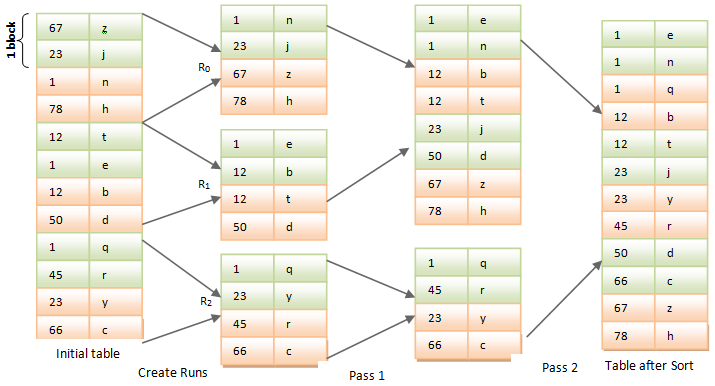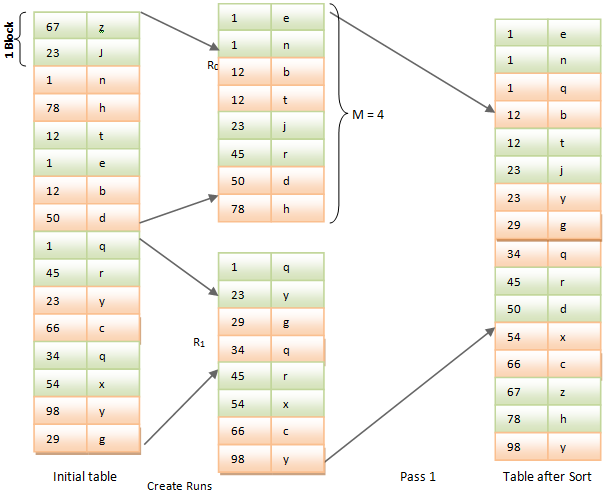Sorting Method in DBMS

It is the technique of storing the records in ascending or descending order of one or more columns. It is useful because, some of the queries will ask us to return sorted records, or in operations like joins will be more efficient in sorted records. All the records are by default sorted based on the primary key column. In addition, we can specify to sort the records based on other columns, as required. Two types of sorting methods are mainly used.

Quick Sort

If a table size is small and can be accommodated into current memory, then quick sort can be used. As the name suggests it is simple and easy method of sorting. In this method a pivot element is identified among the values of the column and values less the pivot element is moved to the left of pivot and greater than pivot elements are moved to the right of the pivot. It takes very less additional space (n log (n)) to sort. It takes only n log (n) time to sort at best case and only n2 time at worst case. But this method is less stable as it can alter the position of two similar records while sorting.

Merge Sort

For the larger tables which cannot be accommodated in the current memory, this type of sorting is used. It has better performance compared to quick sort. Let us see how this sort can be done.

Structure of DBMS

Suppose each block can hold two records and memory can hold up to 2 blocks. That means memory cannot hold all the records of large tables; it can hold up to 4 records only. Suppose Initial table has 12 records with two records in each block.  When merge sort is applied, the records are grouped into 3 with two blocks each. Each block is merged at pass 1 and sorted for the pass 2, where it again merged and sorted. At the last stage blocks at pass 2 are merged and sorted to give the final result. This is how a merge sort works.Suppose a memory can hold M blocks and N (its 3 passes in our case above R0 to R2) be the number of initial runs. When a merge sort is initiated, it continuously reads the M blocks of records, sorts them and stores it as a run Ri. It reads and sorts the blocks N times and creates RN runs.
In our example above, memory can hold 2 blocks of data. When merge sort is initiated, it read 2 blocks of data each to sort and then stored as run from R0 to R2 creating 3 runs.

Now consider the case

N<M

In this case it uses N memory blocks to store each runs, and one for final output. i.e.; suppose block size M = 4 and total number of records in initial table is 16, and each block can hold only 2 records, then we will have 2 runs at the initial stage. i.e.; N<M → 2<4. Hence it will use 2 memory blocks the runs and one for the output.

Data IndependenceIt will repeat the following steps until N blocks are sorted and emptied

• Pick the smallest record from the input runs and write to the output block. . It then removes that smallest record from the respective run block. i.e.; it searches for the smallest record in both the runs above and stores it into output block –as a first step (1, e) is stored in the output block and is removed from R0.
• If the output block is full, then update the disk and empty the output block.

N>=M

In this case we have to merge the blocks many times. M-1 runs are merged in each pass and sorted. Each merge will reduce the number of runs by M-1.

In our First example above,
Number of blocks that a memory can hold (M) = 2
Number of initial Runs (N) = 3, starting from zero to two.
Hence number of merge passes = M-1 = 1 → R1 → R0 and R1 are merged and sorted. In the second block only R2 is left and is sorted.
In each pass, merge reduces the number of blocks by = M-1 = 1 → 2 since count starts from zero.

This is how merge sort works. Let us see the cost of the query in this method.

Total number of Seeks

• Initially it requires one seeks to read the records and one seek to store the records in each run. Hence it requires 2B/M seeks for each run. i.e.; it has to search the records in the block for smallest record and it has to store it in the memory block. Hence it requires one seeks each for reading and writing the records.
• Number of merge pass seek = 2B/NB where NB is the number of blocks read and stored.
• Hence total number of seek = 2B/M + (B/NB)(2logM-1(B/M)-1)